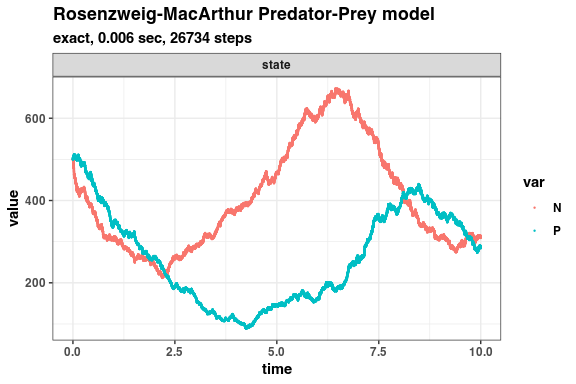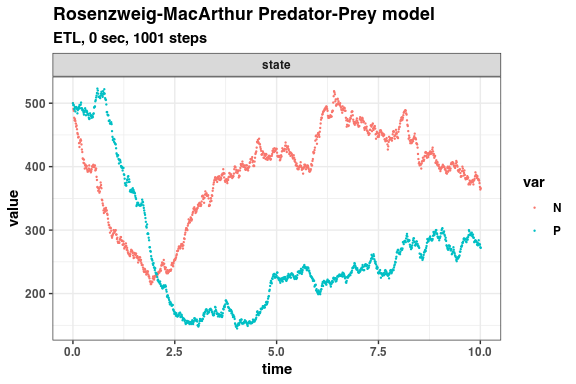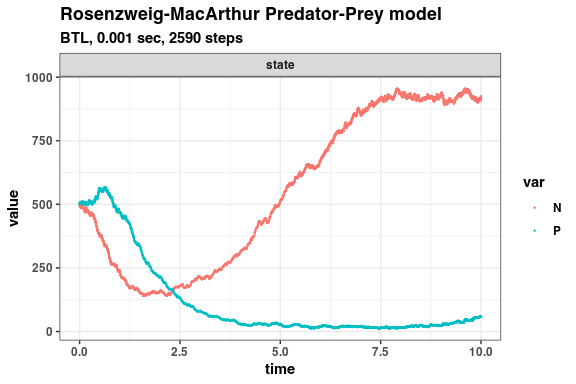# Rosenzweig-MacArthur Predator-Prey model (Pineda-Krch et al., 2007)

Rosenzweig-MacArthur predator-prey model (Pineda-Krch et al., 2007, Pineda-Krch, 2008)

dN/dt = r(1-N/K - alpha/(1+wN))NP
dP/dt = c*alpha/(1+wN))NP

This model has five reactions with the following per capita rates,

prey birth:     b
prey death:     d+(b-d)N/K
predation:      alpha/(1+wN)
predator birth: c*alpha/(1+wN)N
predator death: g

Propensity functions:

a1 = b * N
a2 = (d+(b-d)N/K) * N
a3 = alpha/(1+wN) * N * P
a4 = c*alpha/(1+wN) * N * P
a5 = g * P

Define parameters

library(GillespieSSA2)
sim_name <- "Rosenzweig-MacArthur Predator-Prey model"
params <- c(
b = 2,
d = 1,
K = 1000,
alpha = 0.005,
w = 0.0025,
c = 2,
g = 2
)
final_time <- 10
initial_state  <- c(N = 500, P = 500)

Define reactions

reactions <- list(
reaction("b * N", c(N = +1)),
reaction("(d + (b - d) * N / K) * N", c(N = -1)),
reaction("alpha / (1 + w * N) * N * P", c(N = -1)),
reaction("c * alpha / ( 1 + w * N) * N * P", c(P = +1)),
reaction("g * P", c(P = -1))
)

Run simulations with the Exact method

set.seed(1)
out <- ssa(
initial_state = initial_state,
reactions = reactions,
params = params,
final_time = final_time,
method = ssa_exact(),
sim_name = sim_name
)
autoplot.ssa(out)Run simulations with the Explict tau-leap method

set.seed(1)
out <- ssa(
initial_state = initial_state,
reactions = reactions,
params = params,
final_time = final_time,
method = ssa_etl(tau = .01),
sim_name = sim_name
)
autoplot.ssa(out)Run simulations with the Binomial tau-leap method

set.seed(1)
out <- ssa(
initial_state = initial_state,
reactions = reactions,
params = params,
final_time = final_time,
method = ssa_btl(),
sim_name = sim_name
)
autoplot.ssa(out)